# CBSE Worksheet for chapter-2 Number System class 9

## Find CBSE Worksheet for chapter-2 Number System class 9

CLASS-9

BOARD: CBSE

Mathematic Worksheet - 2

TOPIC: Number System

For other CBSE Worksheet for class 9 Mathematic check out main page of Physics Wallah.

OBJECTIVE:

1. Which of the following is not rational number?
1. √2
2. √4
3. √9
4. √16
2. The number(3-√3)/(3+√3)is
1. Rational
2. irrational
3. both
4. can’t say
3. The exponential form of is
1.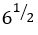2.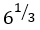3.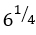4. 6
4. The rational form of 2.7435 is
1. (27161@.)/9999
2. 27/99
3. 27161/9900
4. 27161/9000
5. 5.2 is equal to
1. 45/9
2. 46/9
3. 47/9
4. none

#### SUBJECTIVE :

1. Prove that √3+√5 is a rational number.
2. Find one irrational number between two rational 0.1111….and 0.1100….
3. Represent √8.3 on number line.
4. Prove that (√2+√5)is an irrational number.
5. Multiply .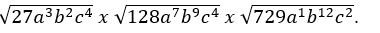6. Simplify 0.4 + 0.18.

OBJECTIVE

1. IF x=3 + √8 and y= 3 -√8 then, =1/x2 +1/y2
1. -34
2. 34
3. 12
4. -12
2. If (3+√7)/(3-√7) = a +b√7 then (a,b)=
1. (8,-3)
2. (-8,-3)
3. (-8, 3)
4. (8, 30)
3. If x= (3+√7)/(3-√7) and y=1 the values of (x-y)/(x-3y)is
1. 5/(√6-4)
2. 5/√(6+4)
3. (√6-4)/5
4. (√6-4)/5
4. The value of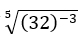is
1. a) 1/8
2. b) 1/16
3. c) 1/32
4. d) none
5. Simplify: 2/(√5+√3)+1/(√3+√2)-3/(√5+√2)
1. 1
2. 0
3. 10
4. 100
6. 25x 2x=1. 0
2. -1
3. 1/2
4. 1
7.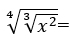1. x
2. x1/2
3. x1/3
4. x1/6

#### SUBJECTIVE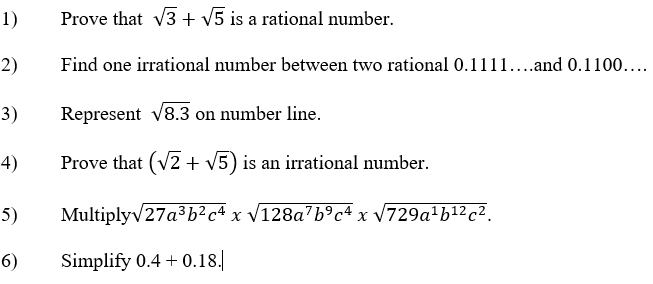1. b
2. d
3. d
4. a
5. b
6. d
7. d

3)(12+56√10)/3
4)

1. 145,
2. 283,
3. 17

4) y(9⁄4)/x(5⁄6)